Select Page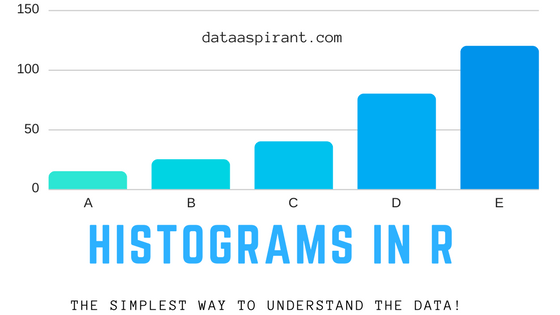Histogram in R

## How to create histograms in R

To start off with analysis on any data set, we plot histograms. Knowing the data set involves details about the distribution of the data and histogram is the most obvious way to understand it.

Besides being a visual representation in an intuitive manner. It gives an overview of how the values are spread.

We come across many depictions of data using histograms in our day to day life. For example, the distribution of marks in a class can be best represented using a histogram and so does the age distribution in an organization.

The good thing about histograms is that it can visualize a large amount of data in a single figure and convey lots of information.

It is quite easy to spot the median and mode by looking at histograms. A histogram can also indicate possible outliers and gaps in data. Thus a single figure can help know a lot about data.

So in this article, we are going implement different kinds of histograms. Starting with the basic histogram and to customize it to a great extended.

Before we drive further let’s look at the table of content for this article.

• Basics of Histogram
• Implementing different kinds of Histograms

## Basics of Histogram

A histogram consists of bars and is made for one variable at a time. That’s why knowledge of plotting a histogram is the foundation of univariate descriptive analytics.

To plot a histogram, we use one of the axis as the count or frequency of values and another axis as the range of values divided into buckets.Let’s jump to plotting a few histograms in R.

## Implementing different kinds of Histograms

I will work on two different datasets and cite examples from them. The first data is the AirPassengers data.

This data is a time series denoting monthly totals of international airline passengers. There are 144 values from 1949 to 1960.

Let us see how the data looks like. The plot() function creates a plot of the time series.

#### Plot Air Passengers DataHistogram for Air Passengers Data with Time

Some patterns are inherently visible in the time series. There are trends and seasonality component. The plot clearly shows how the values gradually increase from 100 to 600 due to increasing trend with a repeating seasonality pattern across years.

We can now use the built-in function hist() to plot histogram of the series in R

#### Histogram for Air Passengers Data with Frequency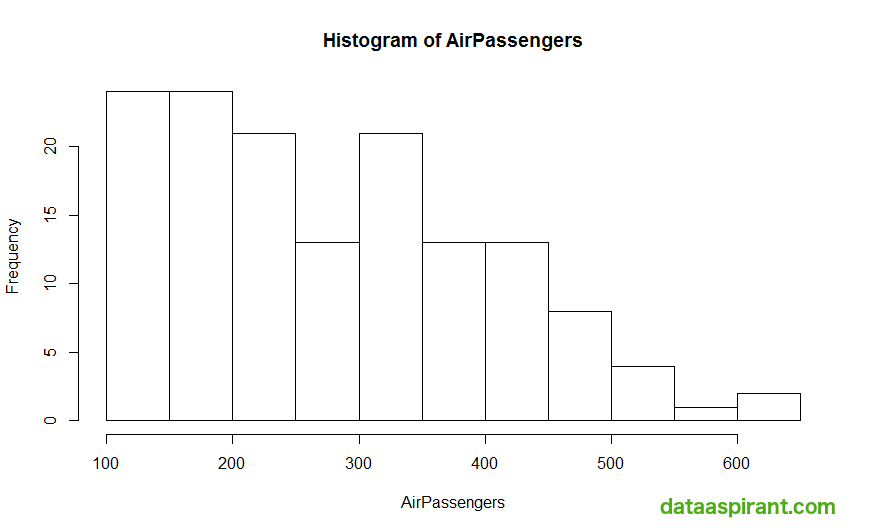Histogram for Air Passengers Data with Frequency

This plot is indicative of a histogram for time series data. The bars represent the range of values and their height indicates the frequency.

The data shows that most numbers of passengers per month have been between 100-150 and 150-200 followed by the second highest frequency in the range 200-250 and 300-350.

Since it is a time series with a gradual seasonality and trend, most of the values are towards the lower end of the spectrum.

That is why the histogram shows a decreasing trend as the values increases. Had it been a time series with a decreasing trend, the bars would have been in increasing order of the number of Air Passengers.

Let’s get into the game

The Air Passengers data was a single variable data. I will now use the iris dataset to help understand more about histograms.

The histogram can plot only one variable at a time. For plotting features of the iris dataset, the \$ notation is used to specify the specific variable I start with plotting the petal length.

#### Petal Length in Distribution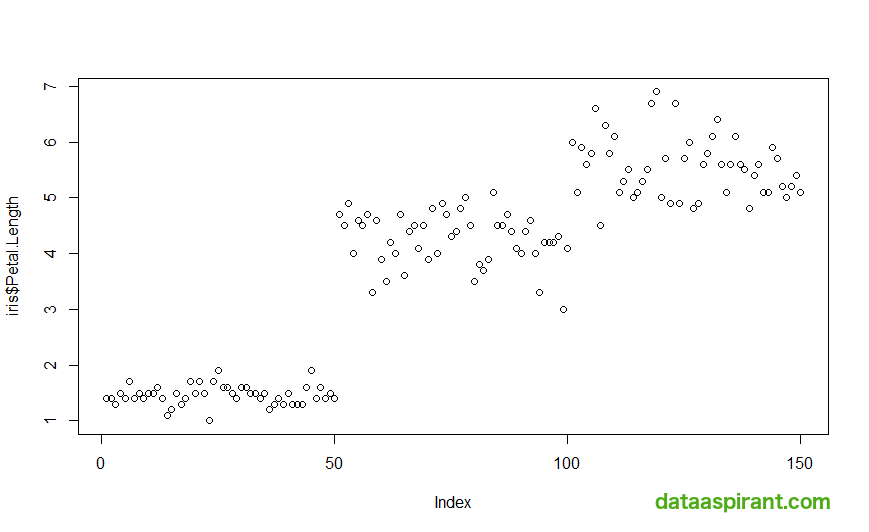Petal length is distributed

The data shows a clear demarcation of three clusters. The clusters have values from 1-2, 3-5 and 5-7 respectively. Let’s make the histogram to get additional insights

#### Histogram for iris petal length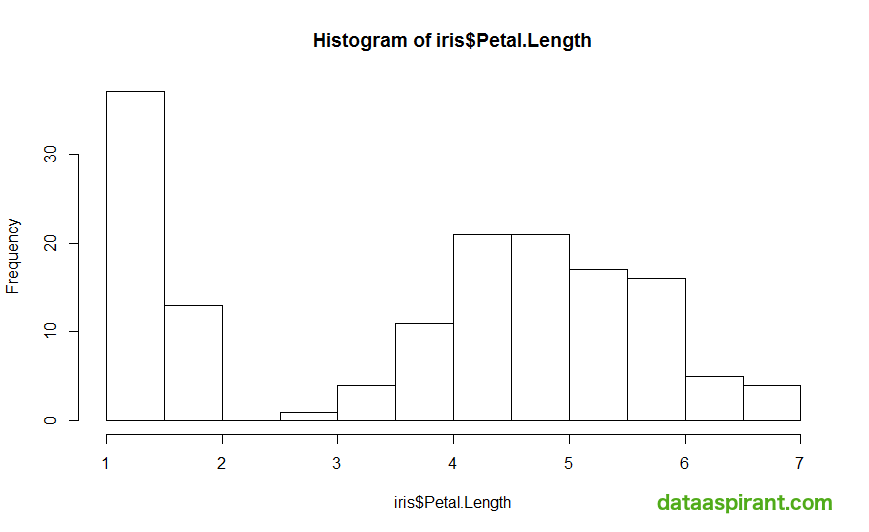Histogram for iris petal length

There are a lot of values in the range 1-1.5 and a small number of values between 1.5-2. This range corresponds to the first cluster. The second and third clusters somewhat overlap. This can be seen from the rest of the values.

There is no gap and the maximum number of values lie between 4-5. There are a few values in the range 2.5-3.5 and beyond 6 which can belong to cluster 2 and cluster 3 respectively.

The iris dataset also contains a non-numeric feature – Species. The plot function can give the count of each species. For this feature, the hist() function will give an error.

#### Distribution of Species in Iris data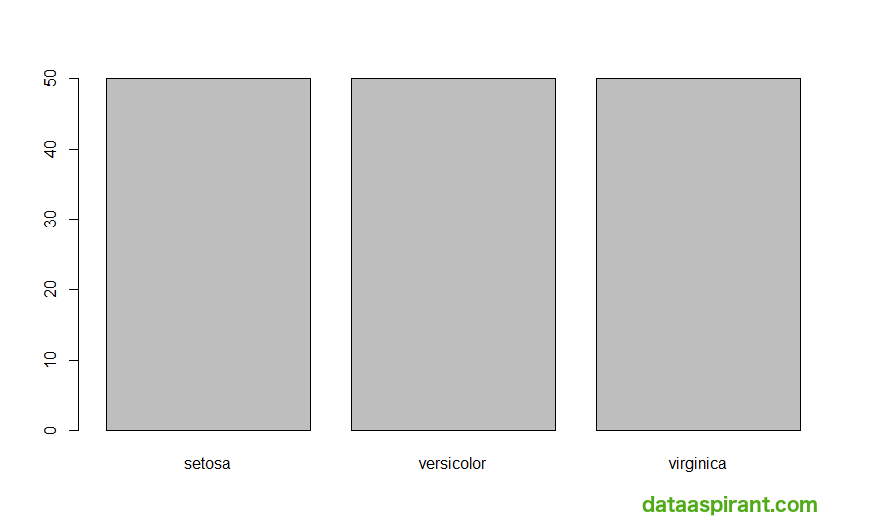Distribution or Species in iris data

Output:

As apparent as it is, the plot function provides a count of all the values and thus histogram is not used to show the distribution of non-numeric features.

However, the hist() function in R is very rich. You can specify a lot of parameters. The important ones are specifying the axis, title, and color of the histogram. You can also specify limits to the axis and change bin size

Adding cheery to the cake – parameters for hist() function

Before looking at the commonly used parameters for a histogram, we first use the help function.

#### Documentation Output

The hist function contains about 20+ parameters. We first look at the way to describe the title to the plot and the labels to x and y-axis. The parameter main=”” specifies the title and labels to the axis are plotted by xlab and ylab for x-axis and y-axis respectively. I will go step by step and set these values for iris petal length featureHistogram for petal length with labels

The next step is to add colors and border. The border parameter and col parameter can be used to set this.Histogram for petal length with Labels and Colors

Now it looks a little lovelier. To make the y-axis indexes more readable, we can rotate it using the las function. las is 0 by default and is parallel to axis. It can be 1 for projecting horizontally. las=, 2 makes x-axis indexes perpendicular to axis along with y axis indexes 3 for y axis indexes parallel to axis while keeping x axis perpendicular.Histogram with y-axis indexes horizontal

We now change the bars. hist() function allows setting the limits of the axis using the xlim and ylim parameters. We can also set the length of the bars using the breaks parameterHistogram with axis limits

Keep in mind that setting the breaks can be done by keeping breaks as a vector as well. Another parameter include.lowest also goes with the breaks when it is a vector. When this parameter is set to true, setting right = FALSE or TRUE will keep all values equal to breaks values in the left bars or right bars respectively.

Additionally, there are two types are histograms. This one is the common one which plots the frequencies of values. A similar plot can be made using probability by setting the freq=FALSE or probability=TRUE parameters.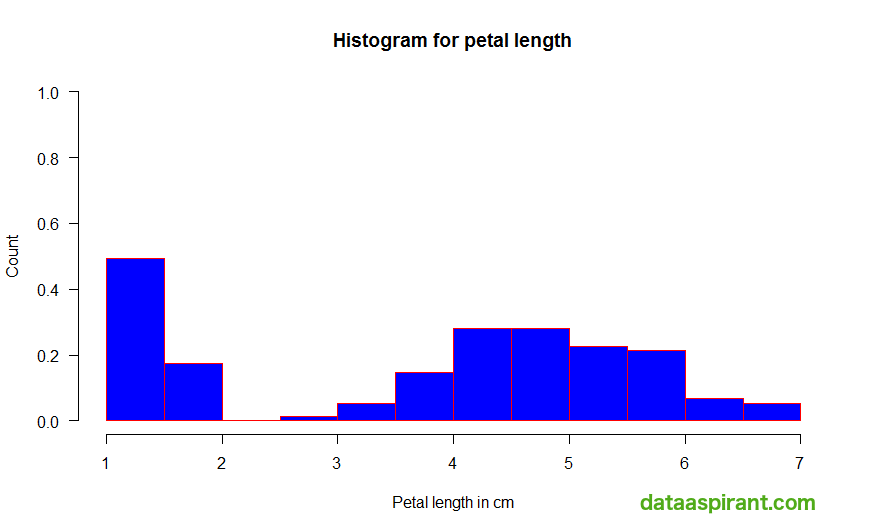Probabilistic Plot

The hist() function also provides color shading. The density and angle parameters come in the picture for this. We can set the color density in lines per inch and the angle of the lines. Let’s try an example with density set to 50 and an angle of 60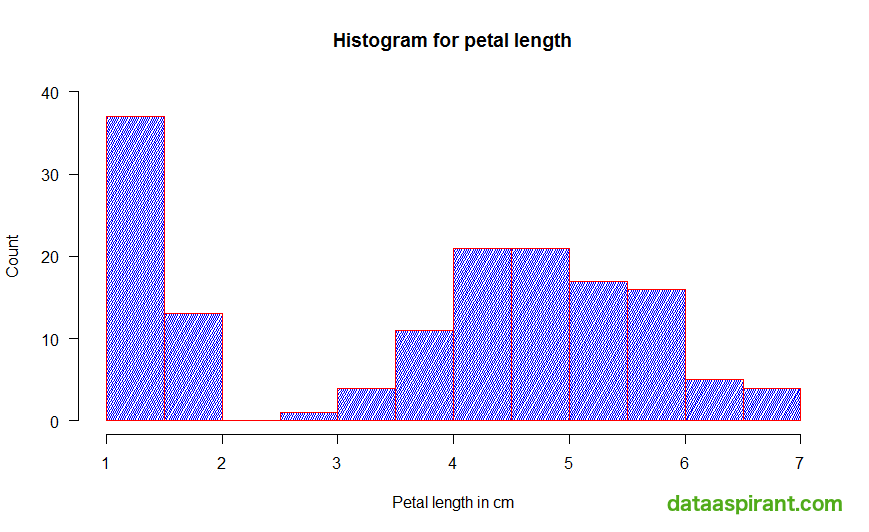Histogram with Color Density in Lines

Additionally, it may not even be necessary to get a plot. We can get the output in our console. The plot parameter, when set to FALSE, gives us the relevant. Let’s try it out

#### Console Output

The final parameter which I am going to explore is the labels parameter. To get more clarity on our plots, the labels parameter writes the exact value the bar is representing when set to TRUE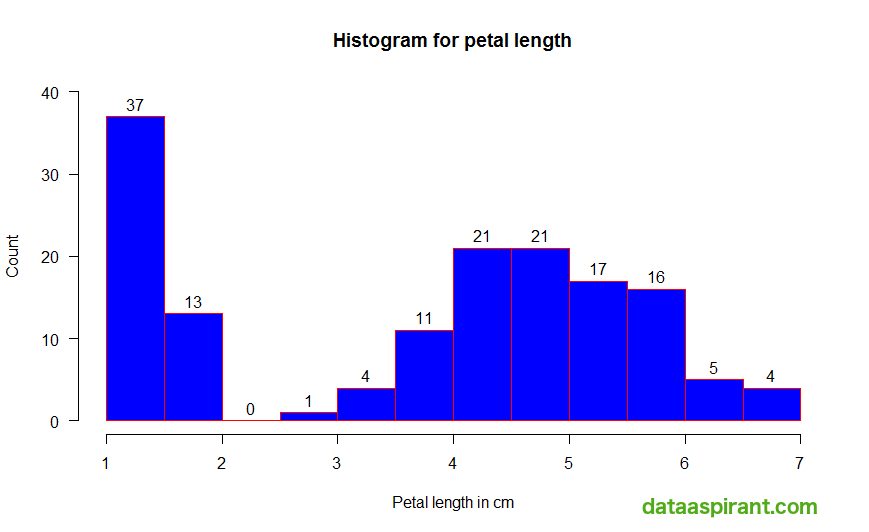Drawing Labels on Bars

Isn’t this a lot of features a built-in histogram function can do? With histograms, one can know so much about the data and make the plots look good as well. For practice, here is the complete R code used in this article.

You can get this post complete code on our Github account.